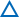欢迎访问贵阳成考网！ 本本网站仅为贵州成人高考考生提供福建成人高考专升本、高起专、高升本、贵州专升本报名咨询以及贵州成人高考考前辅导服务，官方信息以贵州招生考试院www.eaagz.org.cn为准。
• 0851-85985067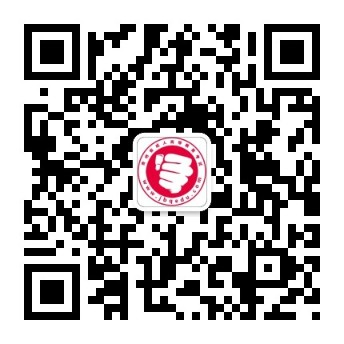# 2022年贵阳成人高考高起本《数学(文)》章节难点习题(2)

2022-05-17 09:12:10 来源：贵阳成考网

【导读】贵阳成考网为帮助想要报考2022贵州省成考的同学更好的复习，特整理了2022年贵阳成人高考高起本《数学(文)》章节难点习题(2)以供考生参考，具体如下：

2022年贵阳成人高考高起本《数学(文)》章节难点习题(2)

一、选择题

1.(★★★★)函数f(x)=x|x+a|+b是奇函数的充要条件是( )

A.ab=0 B.a+b=0 C.a=b D.a2+b2=0

2.(★★★★)“a=1”是函数y=cos2ax-sin2ax的最小正周期为“π”的( )

A.充分不必要条件 B.必要不充分条件

C.充要条件 D.既非充分条件也不是必要条件

二、填空题

3.(★★★★)a=3是直线ax+2y+3a=0和直线3x+(a-1)y=a-7平行且不重合的_________.

4.(★★★★)命题A：两曲线F(x,y)=0和G(x,y)=0相交于点P(x0.y0),命题B：曲线F(x,y)+λG(x,y)=0(λ为常数)过点P(x0.y0),则A是B的__________条件.

三、解答题

5.(★★★★★)设α，β是方程x2-ax+b=0的两个实根，试分析a>2且b>1是两根α、β均大于1的什么条件?

6.(★★★★★)已知数列{an}、{bn}满足：bn= ,求证：数列{an}成等差数列的充要条件是数列{bn}也是等差数列.

7.(★★★★★)已知抛物线C：y=-x2+mx-1和点A(3.0)，B(0.3)，求抛物线C与线段AB有两个不同交点的充要条件.

8.(★★★★★)p:-2

参考答案

难点磁场

证明：(1)充分性：由韦达定理，得|b|=|α·β|=|α|·|β|<2×2=4.

设f(x)=x2+ax+b,则f(x)的图象是开口向上的抛物线.

又|α|<2.|β|<2.∴f(±2)>0.

即有 4+b>2a>-(4+b)

又|b|<4 4+b>0 2|a|<4+b

(2)必要性：

由2|a|<4+b f(±2)>0且f(x)的图象是开口向上的抛物线.

∴方程f(x)=0的两根α，β同在(-2.2)内或无实根.

∵α，β是方程f(x)=0的实根，

∴α，β同在(-2.2)内，即|α|<2且|β|<2.

歼灭难点训练

一、1.解析：若a2+b2=0.即a=b=0.此时f(-x)=(-x)|x+0|+0=-x·|x|=-(x|x+0|+b)

=-(x|x+a|+b)=-f(x).

∴a2+b2=0是f(x)为奇函数的充分条件，又若f(x)=x|x+a|+b是奇函数，即f(-x)=

(-x)|(-x)+a|+b=-f(x),则必有a=b=0.即a2+b2=0.

∴a2+b2=0是f(x)为奇函数的必要条件.

答案：D

2.解析：若a=1.则y=cos2x-sin2x=cos2x,此时y的最小正周期为π.故a=1是充分条件，反过来，由y=cos2ax-sin2ax=cos2ax.故函数y的最小正周期为π,则a=±1.故a=1不是必要条件.

答案：A

二、3.解析：当a=3时，直线l1:3x+2y+9=0;直线l2:3x+2y+4=0.∵l1与l2的A1∶A2=B1∶B2=1∶1.而C1∶C2=9∶4≠1.即C1≠C2.∴a=3 l1∥l2.

答案：充要条件

4.解析：若P(x0.y0)是F(x,y)=0和G(x,y)=0的交点，则F(x0.y0)+λG(x0.y0)=0.即F(x,y)+λG(x,y)=0.过P(x0.y0);反之不成立.

答案：充分不必要

三、5.解：根据韦达定理得a=α+β,b=αβ.判定的条件是p: 结论是q: (注意p中a、b满足的前提是Δ=a2-4b≥0)

(1)由 ，得a=α+β>2.b=αβ>1.∴q p

(2)为证明p q,可以举出反例：取α=4.β= ,它满足a=α+β=4+ >2.b=αβ=4× =2>1.但q不成立.

综上讨论可知a>2.b>1是α>1.β>1的必要但不充分条件.

6.证明：①必要性：

设{an}成等差数列，公差为d,∵{an}成等差数列.

从而bn+1-bn=a1+n· d-a1-(n-1) d= d为常数.

故{bn}是等差数列，公差为 d.

②充分性:

设{bn}是等差数列，公差为d′,则bn=(n-1)d′

∵bn(1+2+…+n)=a1+2a2+…+nan ①

bn-1(1+2+…+n-1)=a1+2a2+…+(n-1)an ②

①-②得：nan= bn-1

∴an= ,从而得an+1-an= d′为常数，故{an}是等差数列.

综上所述，数列{an}成等差数列的充要条件是数列{bn}也是等差数列.

7.解：①必要性：

由已知得，线段AB的方程为y=-x+3(0≤x≤3)

由于抛物线C和线段AB有两个不同的交点，

所以方程组 *有两个不同的实数解.

消元得：x2-(m+1)x+4=0(0≤x≤3)

设f(x)=x2-(m+1)x+4.则有

②充分性：

当3

x1= >0

∴方程x2-(m+1)x+4=0有两个不等的实根x1.x2.且0

因此，抛物线y=-x2+mx-1和线段AB有两个不同交点的充要条件3

8.解：若关于x的方程x2+mx+n=0有2个小于1的正根，设为x1.x2.

则0

根据韦达定理： 有-2

反之，取m=- <0

方程x2+mx+n=0无实根，所以p q

综上所述，p是q的必要不充分条件

— —成考服务— —

1.成考咨询在线老师：【点击咨询

2.贵州成人高考院校专业：【点击查看

3.成人高考报名指导入口：【点击报名

146

(一)由于各方面情况的调整与变化本网提供的考试信息仅供参考，敬请以教育考试院及院校官方公布的正式信息为准。

(二）本网注明信息来源为其他媒体的稿件均为转载体，免费转载出于非商业性学习目的，版权归原作者所有。如有内容与版权问题等请与本站联系。联系方式：邮件429504262@qq.com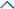上一篇：2022年贵阳成人高考高起本《数学(文)》章节难点习题(1)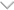下一篇：2022年贵阳成人高考高起本《数学(文)》章节难点习题(3)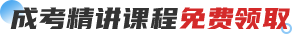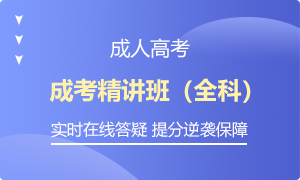2022年成考精讲班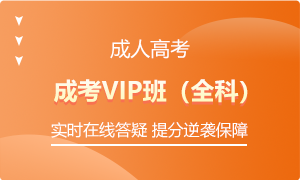2022年成考VIP班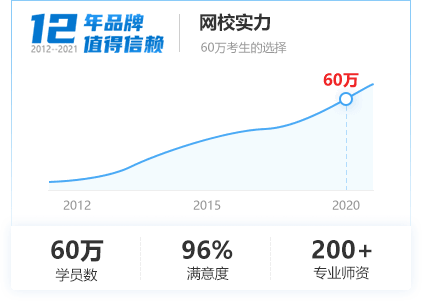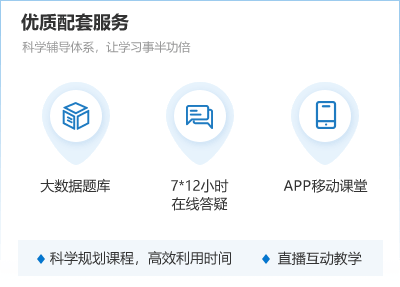7天保障畅学无忧

【成考课程学习中心】

• ## 微信公众号## 免费领取说明

1、培训券可兑换一门视频课程。

2、下载注册APP即可获得400培训券，签到、发帖讨论也可获取培训券。

3、鼓励学习，在线学习也可积累培训券。

4、兑换的课程与购买的为相同课程。

5、课程可在APP或者小程序进行学习。

10/21/2023

• ## 微信公众号## 最新文章